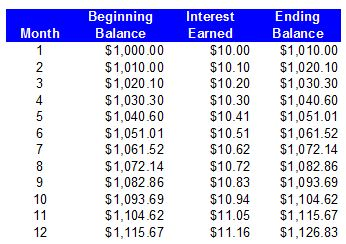# Annual Percentage Yield (APY)

Updated November 4, 2020

## What is Annual Percentage Yield (APY)?

Annual percentage yield (APY) is the rate of interest an investor earns in a year after accounting for the effects of compounding. APY is not the same as annual percentage rate (APR). The formula for APY is:

APY = (1 + (i / n))n - 1

Where:
i = the stated annual interest rate
n = the number of compounding periods in one year

## How Does Annual Percentage Yield (APY) Work?

For example, let's assume you buy a certificate deposit with a 12% stated annual interest rate. If the bank compounds the interest every month (that is, 12 times per year), then using this information and the formula above, the APY on the CD is:

(1 + (0.12 / 12))12 - 1 = 0.12683 or 12.683%

Let's look at it from another angle. Let's assume you put \$1,000 into the 12% CD. Over 12 months, the investment will look like this:The percentage change from \$1,000 to \$1,126.83 is (\$1,126.83 - \$1,000) / \$1,000 = 0.12683 or 12.683%. Even though the bank has advertised a 12% interest rate, your money actually grew by 12.683%.

## Why Does Annual Percentage Yield (APY) Matter?

APY takes compounding into consideration and is thus almost always higher than the stated annual interest rate. It is a useful tool for evaluating the true return on an investment or the true interest rate paid on a loan.

All of our content is verified for accuracy by Paul Tracy and our team of certified financial experts. We pride ourselves on quality, research, and transparency, and we value your feedback. Below you'll find answers to some of the most common reader questions about Annual Percentage Yield (APY).
##### Be the first to ask a question

Paul Tracy - 140Paul has been a respected figure in the financial markets for more than two decades. Prior to starting InvestingAnswers, Paul founded and managed one of the most influential investment research firms in America, with more than 3 million monthly readers.